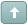Error

 Previous Topic Next Topic
KTP2
#1 Posted : Tuesday, May 4, 2010 4:48:09 PM(UTC)

Groups: Registered, Registered Users, Subscribers
Joined: 2/2/2007(UTC)
Posts: 367

Was thanked: 1 time(s) in 1 post(s)

# Index of Chart Sentiment

Viktor Likhovidov's article, "Index of Chart Sentiment" builds off his previous CandleCode articles. His MetaStock formula for the indicator IndSent - CW requires the formulas he introduced in these previous articles. These formulas are listed below. These formulas are all used by the CandleWeight formula through an Fml() function. It is very important the names are typed exactly the same everywhere you see it. If either spacing or capitalization is off, the referencing formula will give errors.

First create these three formulas. To do so: in MetaStock, go to Tools | Indicator Builder. Click New and enter the name for the formula. Then click in the larger window and enter the actual formula. Click Okay. If the formula was entered with no syntax errors, you will be returned to the Indicator Builder, ready to create the next formula.

Name: body
Formula:
Abs(OPEN-CLOSE)

Name: lshd
Formula:
If(CLOSE>=OPEN, OPEN-LOW, CLOSE-LOW)

Name: ushd
Formula:
If(CLOSE>=OPEN, HIGH-CLOSE, HIGH-OPEN)

After you have created the first three, you can now create the next six formulas. Since they reference the first three, those had to be made first.

Name: ThBot_b
Formula:
BBandBot( Fml( "body") ,55,E,0.5)

Name: ThBot_l
Formula:
BBandBot( Fml( "lshd") ,55,E,0.5)

Name: ThBot_u
Formula:
BBandBot( Fml( "ushd") ,55,E,0.5)

Name: ThTop_b
Formula:
BBandTop( Fml( "body") ,55,E,0.5)

Name: ThTop_l
Formula:
BBandTop( Fml( "lshd") ,55,E,0.5)

Name: ThTop_u
Formula:
BBandTop( Fml( "ushd") ,55,E,0.5)

The CandleWeight formula can now be created. After it is entered, you can create the IndSent - CW formula.

Name: CandleWeight
Formula:

`If(C=O,1,0)*If(Fml("ushd") >= Fml("lshd"),64,-64)+If(C=O,0,1)*If(C>O,1,-1)*(If(Fml("body")<= Fml("ThBot_b"),80,0)+If( Fml("body")> Fml("ThBot_b") AND Fml("body")<=Fml("ThBot_b"),96,0)+If(Fml("body")> Fml("ThTop_b"),112,0))+If(C>=O,-4,4)*(If(Fml("lshd")=0,3,0)+If(Fml("lshd")< Fml("ThBot_l") AND Fml("lshd")>0,2,0)+If( Fml("lshd")> Fml("ThBot_l") AND Fml("lshd")<= Fml("ThTop_l") AND Fml("lshd")>0,1,0))+If(C>=O,1,-1)*(If( Fml("ushd")>0 AND Fml("ushd")<= Fml("ThBot_u"),4,0)+If(Fml("ushd")> Fml("ThBot_u") AND Fml("ushd")<=Fml("ThTop_u"),8,0)+If(Fml("ushd")> Fml("ThTop_u"),12,0))`

After plotting the IndSent - CW indicator, you can quickly plot the four lines with the Standard Error Channel line study. Plot it once, right click on it and change the properties to the desired number of errors. Then you can plot it again for the second number of errors. The same chart below used 1.5 and 1 for the number of errors.

 Wanna join the discussion?! Login to your Discussions forum accountor Register a new forum account.Users browsing this topic
Forum Jump
You cannot post new topics in this forum.
You cannot reply to topics in this forum.
You cannot delete your posts in this forum.
You cannot edit your posts in this forum.
You cannot create polls in this forum.
You cannot vote in polls in this forum.